IF IT'S EDUCATION, IT'S ITS
Pathways To Learning... Since 2005
Hong Kong Registered School 566985

# N - nappe to numerical integration - Mathematics Dictionary

A   B   C   D   E   F   G   H   I   J   K   L   M   N   O   P   Q   R   S   T   U   V   W   X   Y   Z

nappe: One of two surfaces of a double cone partitioned by the vertex of the cone.

natural deduction: A particular family of formal systems of inference.

natural logarithm: Logarithm Naturalis. Logarithm with Euler's number, e, as the base.

natural number: 1. the positive integers: 1, 2, 3, ... etc.

2. the non-negative integers: 0, 1, 2, 3, ... etc.

nautical mile: A unit of length that is exactly 1852 metres. Used mostly in navigations at sea or in the air.

Navier-Stokes equation: Partial differential equations which descrbes the motion (velocity) of a fluid.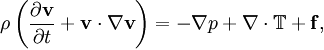n-dimensional: The property of having dimension n.

necessary condition: A sentence which is always true if the specified statement is true, but not necessarily vice versa. A is a necessary condition of B if B is true implies A is true.

negation: The opposite of a mathematical object. A set of all elements which are not elements of the specified set.

negative angle: Angles measured clockwise from a line.

negative binomial distribution: The probability distribution of the number of number of trials before a specified number of successes occur in a series of independent Bernoulli trials with constant probability.

It is to the binomial distirbution as the geometric distribution is to the Bernouli distribution.

Negative Binomial, Geometric - number of trials for a fixed number (k and 1 respectively) of success(es); Binomial, Bernoulli - number of success for a fixed number of (k and 1 respectively) trials

Alternatively, it is to the geometric distribution as the binomial distirbution is to the Bernoulli distirbution.

Negative Bernoulli, Binomial - number of trials/successes for a fixed number of successes/trials; Geometric, Bernoulli - number of trials/successes for 1 success/trial.

negatively associated data: A data set of two variables with a negative correlation.

negative number: A real number with a value strictly less than 0.

negative series: A series consisting of only negative terms.

neighbourhood: A set of points which include all the points within a sphere of radius of any positive value of a specified point.

nephroid: An epicycloid where the radius of the outer (moving) circle is half of that of the inner (fixed) circle.

nested sets: Sets where any two sets where neither is a subset of the other must be mutually exclusive.

net: 1. The opened up and flattened shape from a polyhedron by cutting along edges so that the resulting shape is connected.

2. The remaining amoutn after all deductions are accounted for.

newton: The SI derived unit for the magnitude of a force.

Newton-Cotes rule: A general method of numerical integration which produces a family of formulae approximating a functionwith xi = x0 + h i where h is (xn − x0) / n or equivalently (b − a) / n and wi are the weights.

Newtonian frame of reference: A non-accelerated and non-rotated frame of reference.

Newtonian mechanics: The study of positions (and its time-derivatives) and force through Newton's Law of Motion..

Newton-Raphson method: Also known as Newton's method.

Newton's law of restitution: A law which states that the ratio of the speed two particles approaching each other to that of separating from each other is determined the a quantity called the soefiicient of restitution, CR (sometimes represented as e).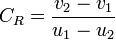Newton's laws of motion: 3 laws about forces and motion of an object that are the basis of Newtonian mechanics:

(I) Without an external force, an object remains in uniform motion (which includes being still)

(2) An external force on an object is proportional to the product of the mass and (the vector quantity of) accelation

(3) For each force (called an action) from an object A on an object B, there is a corresponding force with the same magnitude, but in the opposite direction, from the object B on the object A.

Newton's method: A method for improving the estimate of a root of a continuous function which replacing the current estimate with the x-intercept of the tangent at the current estimate.

Algebraically, a new estimate x1 can be found from an existing estimate x0 by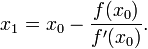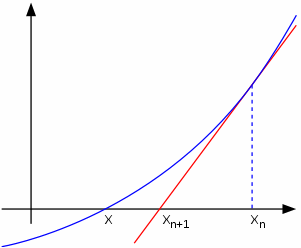Neyman-Pearson lemma: A theorem which provides the best sized critical region for a 2-point hypothesis test using the ratio of the likelihood of the 2 points (values).

n-gon: An n-sided polygon.

nilpotent: A matrix M where Mn = 0 for all positive integers n > 1.

nine-point circle: A circle, that can be constructed from any given triangle, through the 3 midpoints of sides, the 3 points where the height meets the bases and the 3 points between the vertices and orthocentre of the triangle.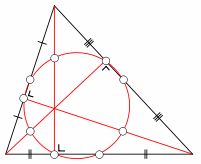noise: Aspects of an observation directly attributed to random error and/or negligible factors, factors not under consideration.

nominal data: A type of categorical data with no obvious ordering that is relevant to the mathematical aspects concerned.

nominal rate: An (interest) stated/given, i.e. the rate based on which calculations are made. This sometimes differ from the actual rate, especially when calculations are based in intervals different from that the rate is based on, even if the sum of the intervals is the same.

nomogram: A type of calculation tool for estimates of values of specific functions.

nonagon: A 9-sided polygon. Also known as an enneagon. The prefix non- is based in Latin while ennea- is Greek.

non-convex: Flat or concave.

nondenumerable: An uncountable set, i.e. a set which is not bijective with the natural numbers.

non-Euclidean geometry: Any system of geometry not based on (all 5 of the) Euclidean axioms/postulates. e.g. hyperbolic geometry, spherical geometry.

non-invertible matrix: A matrix which can not be inverted, i.e. a matrix with no inverse matrix.

nonlinear: The property of an algebraic expression where the highest order term is of order more than 1.

non-negative: Zero or positive.

nonperiodic decimal: A decimal number whose digits do not repeat, which makes the number an irrational number.

nonperiodic tiling: A pattern of non-overlapping geometric shapes which fills a plane with no gaps between shapes, where patterns are generally repeated through the plane.

nonrepeating decimal: Also known as nonperiodic decimals or non-recurring decimals.

nonsingular matrix: A matrix which has an inverse matrix, also called an invertible matrix.

nonterminating fraction: A (possibly mixed) fraction whose denominator is another nonterminating fraction.

non-trivial solutions: Solutions to a system of equations which is not trivial, i.e. not solutions which are independent of parameters.

norm: A function which assigns a non-negative value to all vectors - the generalisation of the modulus function. A norm ||v|| for the vector v is a function where:

(1) ||v|| =0 for v = 0; ||v|| ≥ 0 for all other v

(2) For a vector v and a scalar k, ||kv|| = |k|.||v||

(3) Triangle inequality - ||u+v|| ≤||u||+||v|| for all u, v

normal: 1. Being perpendicular to a given geometric figure. e.g. normal to a line or a plane

2. The line or vector which is perpendicular to a given geometric figure.

3. having the property of the normal distribution.

normal component: The component of acceration which is perpendicular to the velocity.

normal deviate: A normally distribution random variable measuring the directed difference between the value and the mean, expressed in terms of unit of standard deviation.

normal distribution: Also called a Gaussian distribution. It is the limit of a binomial distribution, as the number of trialstend to infinity. The distribution is specified by two parameters, µ (mean) and σ2 (variance). For a random vairbale X, this is symbolically represented by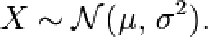where the probability density function is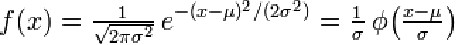The curve for the probability density function is "bell-shaped", which explains the common class="d-title" name of the bell curve. For any normal distribution, roughly 68% of values lie within 1 standard deviation of the mean, roughly 95% within 2 standard deviations of the mean and roughly 99.8% within 3 standard deviations of the mean, as shown in the diagram below: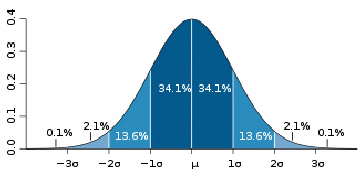normal section: A cross section of a geometric figure with a plane which contains the normal of a point to the face of the geometric figure.

normed space: A vector space equipped with a norm.

NP complete: An NP problem that is also NP-Hard.

NP problem: Nondeterministic Polynomial time decision problems - decision problems whose answer can be verified in polynomial time by a turing machine.

nth root: A number which, when raised to the power of n, equals to the specified number of which we take the nthroot.

n-tuple: An ordered set with n members.

nuisance parameter: An parameter not of interest, but which must be included in the model in order to analyse those parameters which are of interests.

null angle: The angle formed by two coincident lines sharing the same endpoints, i.e. 0o.

null hypothesis: The hypothesis assumed, for which calculations of probabilities in hypothesis testing are based on. It continues to be the assumption unless the probability of an observation made is smaller than the significanct level if the null hypothesis is right. In which case, we reject the null hypothesis.

nullity: The dimension of the kernel of a morphism, mapping, homomorphism, linear transformation etc.

null matrix: A matrix whose entries are all 0. It is the additive identity of matrix addition, it is also known as the zero matrix.

null sequence: A sequence whose limit is zero.

null set: Also known as the empty set, it is the unique set that contains no elements.

null vector: Also known as a zero vector.

number line: A graphical representation of the structure (i.e. total order) of subsets of the real numbers (which include the set of real numbers itself) in the form of a line.

number sieve: A method for finding prime factors of a number by eliminating all multiples (less than the specified number) of the lowest number not yet eliminated. Starting from the positive integers greater than 1, the lowest number not eleiminated in each "pass", is a prime factor of the specified number.

As numbers getting progressively eliminated (sieving), all prime factors will eventually be found.

number theory: The study of number properties in mathematics.

numerator: The number or expression above the horizontal line (on top) in a fraction. Representing the dividend in the division.

numerical differentiation: The process of approximating of the gradient of the tangent of a function at a particular point by the line through 2 points nearby, usually the contact point itself is used as one of the points.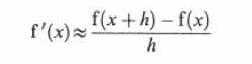numerical equation: An equation with known, numerical coefiicients and constants.

numerical integration: The process of approximating the definite integral of a function in an interval by values of the function with arguments in the interval. More accurate approximations results from more points within the interval being used. The points are usually chosen so that they partition the interval evenly.

A   B   C   D   E   F   G   H   I   J   K   L   M   N   O   P   Q   R   S   T   U   V   W   X   Y   Z

Genius is one percent inspiration and ninety-nine percent perspiration.